# Electronics Module by Sam Kinyera OBWOYA - HTML preview

PLEASE NOTE: This is an HTML preview only and some elements such as links or page numbers may be incorrect.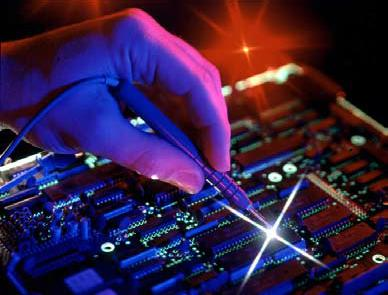Electronics Module

Electronics Module

Prepared by Sam Kinyera OBWOYA

African Virtual university

Université Virtuelle Africaine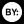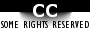African Virtual University

Notice

This document is published under the conditions of the Creative Commons

http://en.wikipedia.org/wiki/Creative_CommonsAfrican Virtual University

I.

Electronics Module _________________________________________ 3

II. Prerequisite Course or Knowledge _____________________________ 3

III. Time ____________________________________________________ 3

IV. Materials _________________________________________________ 3

V. Module Rationale __________________________________________ 3

VI. Content __________________________________________________ 4

6.1 Overview ___________________________________________ 4

6.2 Outline _____________________________________________ 5

6.3 Graphic Organizer _____________________________________ 6

VII. General Objectives _________________________________________ 7

VIII. Specific Learning Objective(s) _________________________________ 7

IX. Pre-assessment ___________________________________________ 9

X. Teaching and Learning Activities ______________________________ 14

XI. Glossary of Key Concepts __________________________________ 149

XII. List of Compulsory Readings _______________________________ 151

XIII. Compiled List of (Optional) Multimedia Resources _______________ 153

XIV. Compiled list of Useful links ________________________________ 155

XV. Synthesis of the Module ___________________________________ 158

XVI. Summative Evaluation _____________________________________ 160

XVII. References _____________________________________________ 163

XVIII. Student Record _________________________________________ 164

XIX. Main Author of the Module _________________________________ 164African Virtual University

I. electronics Module

By Sam Kinyera Obwoya Kyambogo University Uganda

II. Prerequisite Courses or Knowledge

The basic prerequisites for this module are the school physics that one has learnt. In

particular, knowledge of the following courses are essential for one to follow and

understand the module effectively. Some of the prerequisite courses are solids state

physics, electricity and magnetism. As a general requirement, you need the knowledge

of calculus and algebra in mathematics.

III. Time

A total of 120 hours is required for you to complete this module.

IV. Material

The materials required for the module include access to a computer, but more impor-

essential references and multimedia resources. These multimedia are important as in

some cases they serve as virtual lecturers and sources of equipment that can be used

to perform virtual experiments. However, some CD-ROMS will also be available

to supplement the use of internet. Other materials include compulsory readings and

compulsory resources that may be available at nearby bookshops or schools.

V. Module Rationale

This module is intended to provide a basic foundation of physics to students. This

will enable the students to learn the subject matter in order to explain and account for

the principles involved in electronics. The module is structured such that the learner

has to go through the activities as prescribed for maximum attainment. The overall

module will provide the student with basic ideas of what electronics is in terms of the

key components’ behaviours or characteristics and therefore will be enable to teach

most of the school physics effectively.African Virtual University

VI. Content

6.1 Overview

Electronics is the study of the flow of charge through various materials and devices

such as, semiconductors, resistors, inductors, capacitors, nano-structures, and vacuum

tubes. All applications of electronics involve the transmission of power and possibly

information. Although considered to be a theoretical branch of physics, the design and

construction of electronic circuits to solve practical problems is an essential technique

in the fields of electronic engineering and computer engineering.

The study of new semiconductor devices and surrounding technology is sometimes

considered a branch of physics. This module focuses on engineering aspects of

electronics. Other important topics include electronic waste and occupational health

impacts of semiconductor manufacturing.

This course of electronics is intended for students enrolling for pre-service and in-

service students registering for BSc with Education and BEd degrees. As you may be

aware, Electronics forms one the back bone of modern physics. The module has six

units: Diode Circuits; Transistor Circuits; Operational Amplifiers; Digital Circuits;

Data acquisition and Process Control; and Computers and Device Interconnection.

In the first unit/activity i.e. diodes circuits, students are expected to explain charge car-

rier generation, intrinsic and extrinsic semi-conductors, formation and application of

P-N junction, and to design and analyse diode circuits (e.g, power supply circuits).

In the second unit/activity i.e. Transistor circuits, the student is expected to explain

how a Bipolar Junction Transistor (BJT) works; Design and analyse basic BJT cir-

cuits in various configurations (CE, EB, CB); Explain how a junction Field Effect

Transistor (JFET) works ; Design and analyse JFET circuits in both configurations

(CD, CS); Explain how MOSFET works and also be able to Design and analyse

MOSFET circuits.

In unit three the learning outcomes include one being able to explain the construc-

tion of operational amplifier; and to Design, analyse and synthesize operational

amplifier circuits. In unit four, i.e. Digital Circuits, the student is expected to Ma-

nipulate numbers in various bases (2,8,10,16); Apply Boollean algebra in design of

logic circuits; Design, analyse and synthesize logic circuits (multiplexer, decoders,

Schmitt triggers, flip-flops, registers). In unit five the learner will explain the opera-

tion of a transducer in various modes (strain, light, piezo, temp); Explain and apply

transducer signal conditioning processes; and to Apply conditioned signal in digital

form. Finally, in activity six, i.e. Elements of the Microcomputer 8-, 16- or 32Bit

buses, the expected learning will include explaining the systems level components

of a microprocessor.African Virtual University

6.2

Outline

Activity 1

(20 hours)

Diode Circuits Review Energy band theory, The PN Junction and the Diode Effect,

Circuit, Applications of Ordinary Diodes

Activity 2

(30 hours)

Transistor Circuits Bipolar Junction Transistor (BJT) Common Emitter Amplifier,

Common Collector Amplifier, Common Base Amplifier. The Junction Field Effect

Transistor (JFET), JFET Common Source Amplifier, JFET Common Drain Ampli-

fier. The Insulated-Gate Field Effect Transistor. Power MOSFET Circuits. Multiple Transistor Circuits

Activity 3

(10 hours)

Operational Amplifiers Open-Loop Amplifiers, Ideal Amplifier, Approximation

Analysis, Open-Loop Gain

Activity 4

(30 hours)

Digital Circuits Number Systems, Boolean Algebra, Logic Gates, Combinational

Logic. Multiplexers and Decoders. Schmitt Trigger, Two-State Storage Elements,

Latches and Un-Clocked Flip-Flops. Clocked Flip-Flops, Dynamically clocked Flip-

Flops, One-Shot Registers

Activity 5

(20 hours)

Data Acquisition and Process Control Transducers, Signal Conditioning Circuits,

Oscillators, Analogue-to-Digital Conversion

Activity 6

(10 hours)

Computers and Device Interconnection Elements of the Microcomputer 8-, 16- or

32- Bit BusesAfrican Virtual University

6.3

Graphic Organizer

6.2

GRAPHIC ORGANIZER

A. Diode Circuits

Review Energy band theory,

D. Digital Circuits

The PN Junction and the Diode Effect,

Number Systems, Boolean Algebra,

Circuit Appli cations of Or dinary Diodes

Logic Gates, Combinational Logic.

Multiplexers and Decoders. Schmitt Trigger,

B. Transistor Circuits:

Two-State Storage Elements, Latches and Un-Clocked Fli p-Flops.

Bipolar Junction Transistor (BJT)

Clocked Fli p-Flops, Dynamical y clocked Flip-Flops, One-Shot Registers

Comm on Emitter Ampli fier, Comm on

Col ector Ampli fier, Comm on Base Ampli fier.

E. Data Acquisition

and Process Control

The Junction Field Effect Transistor (JFET),

Transducers,

Elect

c roni

n cs

c

JFET Comm on S ource Amplifier, JFET Common Drain Amplifier.

Signal Conditioning Circuits,

The Insulated-Gate Field Effect Transistor.

Power MOSFET Circuits. Multiple Transistor Circuits

Oscil ators,

Analogue-to-Di gital Conversion

C. Operational

Amplifiers

F. Com puters and

Open-Loop Amplifiers,

Device Interconnection

Ideal Ampli fier,

Elements of the Microcomputer 8-, 16- or 32- Bit Buses

Approximation Analysis,

Open-Loop Gain

3African Virtual University

VII. General objective(s)

After completing the module you should be able to

• appreciate and apply basic electronic concepts and circuits

VIII. specific learning objectives

Unit

Learning objective(s)

1. Diode Circuits (20 hours)

Students should be able to

• Review Energy band theory,

• Explain charge carrier generation

• The PN Junction and the Diode

intrinsic and extrinsic semi-conductors

Effect,

• Explain formation and application of

• Circuit, Applications of Ordinary

P-N junction

Diodes.

• Design and analyse diode circuits (e.g,

power supply circuits)

2. Transistor Circuits: (25 hours)

Students should be able to

• Bipolar junction Transistor

• Explain how a Bipolar Junction Tran-

• (BJT);Common Emitter Ampli-

sistor (BJT) works

fier; Common Collector Ampli-

• Design and analyse basic BJT circuits

fier, Common Base Amplifier.

in various configurations (CE, EB,

• The Junction Field Effect Tran-

CB)

sistor (JFET), JFET Common

• Explain how a junction Field Ef-

Source Amplifier, JFET Common

fect Transistor (JFET) works (some

Drain amplifier.

theory)

• The Insulated-Gate Field Effect

• Design and analyse JFET circuits in

Transistor. Power

both configurations (CD, CS)

• MOSFET Circuits, Multiple

• Explain how MOSFET works (theory)

Transistor Circuit

• Design and analyse MOSFET circuits

3. Operational Amplifiers (10 hours)

Students should be able to

• Open loop Amplifiers,

• Explain the construction of operatio-

• Ideal Amplifiers, Apporoxima-

nal amplifier

tion Analysis, Ope-loop Gain.

• Design, analyse and synthesize opera-

tional amplifier circuitsAfrican Virtual University

4. Digital Circuits (30 hours)

Students should be able to

• Number systems, Boolean Alge-

• Manipulate numbers in various bases

bra, Logic Gates,

(2,8,10,16)

• Combinational Logic,

• Apply Boollean algebra in design of

• Multiplexes and decoders, Sch-

logic circuits

mitt Trigger, Two-State storage

• Design, analyse and synthesize logic

elements,

circuits (multiplexer, decoders, Smch-

• Latches and un-clocked flip-

mitt triggers, flip-flops, registers)

flops;

• Dynamically clocked flipiflops,

• One-shot registers

5. Data acquisition and Process

Students should be able to

Control (20 hours)

• Explain the operation of a transducer

• Transducers, Signal Conditioning

in various modes (strain, light, piezo,

• circuits, Oscillators, Analogue-

temp)

to-Digital Conversion

• Explain and apply transducer signal

conditioning processes

• Apply conditioned signal in digital

form

6. Computers and Device Inter-

• Explain the systems level components

connection (15 hours)

of a microprocessor

• Elements of the Microcomputer

8-, 16- or 32- Bit BusesAfrican Virtual University

IX. Pre-assessment

Are you ready to Learn Electronics?

Title of Pre-assessment : ELECTRONICS

Rationale : The pre-assessment is intended to determine how much one remembers

and what one knows about electronics which was done at school and therefore to

orient the mind of the learner of the amount of work expected to be covered during

the course. The pre-assessment is not intended in anyway to discourage the learner,

but rather to motivate one to start the course with a lot of readiness for the challenges

9.1

Self Evaluation Associated With Electronics

1 The resistance of the semiconductor materials in a photoconductive cell varies

with the intensity of incident light.

a. directly

b. inversely

c. exponentially

d. log arithmetically

2 A solar cell operates on the principle of

a. diffusion

b. recombination

c. carrier flow

d. photovoltaic action

3 Which of the following devices has the highest sensitivity?

a. photoconductive cell

b. photovoltaic cell

c. photodiode

d. phototransistor

4 In LED, light is emitted because

a. recombination of charge carriers takes place

b. light falling on the diode gets amplified

c. light gets reflected due to lens action

d. diode gets heated upAfrican Virtual University 0

5 A transistor series voltage regulator is called emitter-follower regulator because

the emitter of the pass transistor follows the voltage.

a. base

b. input

c. output

d. collector

6 A switching voltage regulator can be of the following type:

a. inverting

b. step-up

c. step-down

d. all of the above

7 An ideal voltage regulator has a voltage regulation of

a 0

b. 1

c. 50

d. 100

8 Electronic devices that convert dc power to ac power are called

a inverters

b. rectifiers

c. converters

d. transformers

9 The output of a half-wave rectifier is suitable only for

b. running ac motors

c. running tape-recorders

d. charging batteries

10 When used in a circuit, a Zener diode is always

a. forward biased

b. reverse-biased

c. connected in series

d. troubled by overheatingAfrican Virtual University

11 Zener diodes are used primarily as

a. rectifiers

b. amplifiers

c. oscillators

d. voltage regulators

12 An op-amp shunt regulator differs from the series regulator in the sense that its

control element is connected

a. series with line resistor

b. parallel with line resistor

d. parallel with input voltage

13 The digital systems usually operates on……system.

a. octal

c. binary

d. decimal

14 The cumulative addition of four binary bits (1 + 1 + 1 + 1) gives

a. 1111

b. 111

c. 110

d. 11

15 The result of binary multiplication 111 x 10 is

2

2

a. 1101

b. 0110

c. 1001

d. 1110

16 A FETs have similar properties to

a. PNP transistor

b. NPN transistor

c. thermionic valves

d. Unijunction transistorAfrican Virtual University

17 The voltage gain of a given common-source JFET amplifier depends on its

a. input impedance

b. amplification factor

c. dynamic drain resistance

18 The extremely high input impedance of a MOSFET is primarily due to the

a. absence of its channel

b. negative gate-source voltage

c. depletion of current carriers

d. extremely small leakage current of its gate capacitor

19 The main use of an emitter follower is as:

a. power amplifier

b. impedance matching

c. low-input impedance

d. follower of base signal

20 The smallest of the four h- parameters of a transistor is:

a. hi

b. hr

c. h0

d. hf

1. B

11. D

2. D

12. A

3. D

13. B

4. A

14. B

5. A

15. D

6. D

16. B

7. A

17. D

8. A

18. C

9. B

19. B

10. B

20. CAfrican Virtual University

Pedagogical Comment For Learners

The pre-assessment is intended to determine how much you know of electronic and

to prepare you for the module. The outcome of the pre-assessment will tell you of

what you need to work on and concentrate on more while studying and learning the

module. As you notice, most of the questions contain topics which are normally not

done at school.

At the beginning of the module, the module takes you through the review energy band

theory, which you could have done in solid state physics. Eventually you will learn

about the PN-junction and diode effect, circuit, and applications of ordinary diodes.

The expectation here is that you should be able to explain charge carrier generation

intrinsic and extrinsic semi-conductors, formation and application of P-N junction;

and finally be able to design and analyze diode circuits (e.g. power supply circuits).

For every other activity you go through them with the expectation of achieving the

stated objectives. Accordingly, you are advised to go through each section of the

activity in a chronological order. Where prior knowledge is required, you need to

go first through such topics before proceeding further.

A number of references are referred to throughout the activity. What you need to do

always is to have access to these references. Most of them are on line. Where you do

and keep hard copies. A number of multimedia resources are also included. These

are very useful as they may act as virtual lecturers or sources of virtual laboratory.

You are encouraged to use these multimedia resources all the time.African Virtual University

X. Teaching and learning activities

Activity 1: Diode Circuits

You will require 20 hours to complete this activity. Only basic guidelines are provided

Specific Teaching and Learning Objectives

In this activity you will

(i) Explain charge carrier generation in intrinsic and extrinsic semi-conductors.

(ii) Explain formation and application of P-N junction.

(iii) Design and analyse diode circuits (e.g, power supply circuits.

Summary of the learning activity

This activity includes among others explanation of charge carrier generation, intrinsic

and extrinsic semi-conductors; formation and application of P-N junction and finally

how to design and analyse diode circuits (e.g, power supply circuits)

Complete reference: http://en.wikibooks.org/wiki/Electronics. 3rd October 2007.

Abstract: This is a complete textbook on electronics that deals with among others:

analogue circuits: vacuum tubes; diodes, transistors; amplifiers; operational ampli-

fiers, and analogue multipliers.

Rationale: Each of the topic is presented in very simple form that makes it easier

for one to read through. However, these simply serve to supplement the learning

process.

Reference: http://en.wikipedia.org/wiki/electronics. 5th October 2007.

Abstract: This reading is formed from references obtained from many sites. Their

URLs can be obtained from a soft copy of this reading. Basically all the essential

topics of the course are covered in this reading 2.

Rationale. The reference provide easy reading sources on electronics that a reader

should have no problem using them.African Virtual University

List of relevant MULTIMEDIA resources.

Reference: http://www.educypedia.be/electronics/javacollectors.htm.

Summary: This resource enables one to study Characteristics of NPN transistor.

Rationale: The site gives an elegant simple virtual experiment that one can carry to

study the characteristics of NPN transistor.

Reference: http://server.oersted.dtu.dk/personal/ldn/javalab/Circuit04.html

Summary: The resource is for circuit of a primitive common-emitter (CE) amplifier-

comprising an npn-transistor and external basis-, collector- and load resistors. The

learner will find for a fixed set of component parameters the ranges ofinput voltage

that make the transistor cut off, active or saturated, respectively. In the case of a na-

logue applications the learner will d etermine the differential voltage amplification

of the circuit when the transistor is in the active range. While for d igital applications

one is expected to find the smallest possible current gain (beta) and a corresponding

collector resistance that makes the circuit a functional logical inverter.

Rationale: This resource serves to aid one in learning about npn transistor biasing.

Reference: http://server.oersted.dtu.dk/personal/ldn/javalab/Circuit01.html

Summary: This resource gives a circuit of a Thevenin equivalent with a load in which

power P is delivered to the load.

Rationale: This site provides a useful resource for learning about voltage divider.

Title: Basic circuit analysis

http://ocw.mit.edu/OcwWeb/Electrical-Engineering-and-Computer-Science/6-

002Circuits-and-ElectronicsFall2000/VideoLectures/index.htm.

Abstract: These contain the course lecture slides accompanying video lectures, and

description of live demonstration shown by the instructor.

Title: Diodes

URL: http://jersey.uoregon.edu/~rayfrey/431/lab2_431.pdfhttp://jersey.uoregon.

edu/

Abstract: This site provides practical work V-I characteristics. In addition, the site

provides reading on transistor junctions, transistor switch and saturation etc.

Title: Diode applications

URL: http://morley.eng.ua.edu/G332BW.pdf.

Abstract: Various applications of diodes including power supply, half-wave rectifier,

bridge rectifier, full-wave rectifier with filter etc. are presented.African Virtual University

Detailed Description of the Activity (Main Theoretical Elements)

Activity 1.1

Review of energy band theory

Key Concepts about energy band theory

The key concepts one learns about energy band theory under solid state physics

are:

(i) That the available energy states form what we call bands.

(ii) That in insulators the electrons in the valence band are separated by a large

gap called forbidden energy gap from the conduction band.

(iii) That insulators have empty conduction band, but a full valence band.

(iv) That in conductors like metals the valence band overlaps the conduction band,

and therefore, there is no structure to establish holes. The total current in such

conductors is simply a flow of electrons.

(v) That a semiconductor material is one whose electrical properties lie in between

those of insulators and good conductors. In terms of energy band, semiconduc-

tors can be defined as those materials which have almost an empty conduction

band and almost filled valence band. There is a small enough gap between

the valence and conduction bands that thermal or other excitations can bridge

the gap. With such a small gap, the presence of a small percentage of a doping

material can increase conductivity dramatically.

(vi) That the outermost electrons of an atom i.e. those in the shell furthermost

from the nucleus are called valence electrons and have the highest energy or

least binding energy.

(vii)

The band of energy occupied by the valence electrons is called the va-

lence band and is the highest occupied band. It may be completely filled or

partially filled with electrons but never empty .

Important instruction

1. For each task that you undertake you must make short notes using some of the

references that are given to you and including those that you can have access

to.

2. Use available electronic text books and other references e.g. http://hyperphysics.

phy-astr.gsu.edu; to

of: energy band, valence band, conduction band, energy gap, and Fermi le-

vel.

(b) Make short notes about each one.African Virtual University

(c) Distinguish between conductors, semiconductors, and insulators.

(d) Sketch side by side, diagrams showing energy bands in conductors, semicon-

ductors and insulator.

(e) Explain what is meant by intrinsic semiconductors; and binding energy.

Learning points 1.1

Under this section you learn:

(i) That the position of the Fermi level in relation to the conduction band is an

important factor in determining electrical properties of materials.

(ii) That the large energy gap between the valence bands in an insulator says that

at ordinary temperatures, no electrons can reach the conduction band.

(iii) That in semiconductors, the band gap is small enough that thermal energy

can bridge the gap for a small fraction of electrons. In conductors, there is no

band gap since the valence band overlaps the conduction band.

(iv) That for intrinsic semiconductors like silicon and germanium, the Fermi level

is essentially halfway between the valence and conduction bands. Although

no conduction occurs at 0 K, at higher temperatures the width of the forbid-

den energy bands is decreased and a finite number of electrons can reach the

conduction band and provide some current. Conductivity of a semiconductor

increases with temperature.

(v) That an intrinsic semiconductor is one which is made of semiconductor ma-

terial in its extremely pure form. Alternatively an intrinsic semiconductor

may be defined as one in which the number of conduction electrons is equal

to the number of holes.

Activity 1.2

Origin of charge carriers

We can develop this concept by reminding ourselves of atomic structure and what

valence electrons are.

In order to understand the origin of charge carrires, carry out the following tasks:

(a) Read and write short notes on what is meant by atomic structure

(b) Sketch the atomic structures of germanium (Ge) and silicon (Si). In addition

to this, it is also a good practice to draw the electronic distribution of an ele-

ment for comparison with the atomic structure. For example the electronic

distribution of silicon atom (Z = 14) is shown in Fig. 1.1.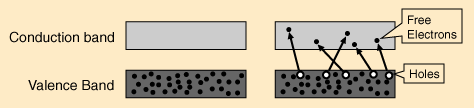African Virtual University

Conduc tion band

Va lenc e band

2nd band

1st band

Figure 1.1. Electronic distribution of silicon atom

(c) The atomic structure for Ge you have drawn should show that germanium

consists of:

(i) a central nucleus which is positively charged, and

(ii) four electrons in the outermost orbit. These four electrons are called

valence electrons. This is the same as the number of electrons in the valence

band.

1.2.2 Intrinsic Semiconductors: Electrons and Holes

Common examples of semiconductors are germanium and silicon which have forbid-

den energy gaps of 0.72 eV and 1.1 eV respectively. At temperatures above 00K some

electrons are excited to conduction band leaving behind positively-charged holes in

the valence band as shown in Fig. 1.2. Note that only valence and conduction bands

are shown since the lower filled bands are not of any consequence.

Figure 1.2. Electrons excited to conduction band leaving positively charged holes in valence band If an external voltage, is applied across the silicon, conduction electrons move to

the anode, while the holes in the valence band move to the cathode as shown in Fig.

1.3. Hence semiconductor current consists of movement of electrons and holes in

opposite directions.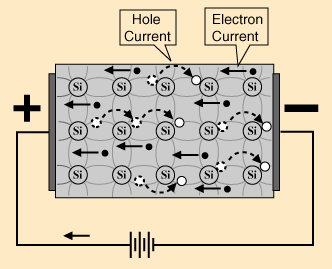African Virtual University

Figure 1.3. Conduction electrons move to the anode(+), while holes in the valence

band move to the cathode(-) when voltage is applied across them.

The conduction electron-hole pairs formed constitute the charge carriers. The num-

ber, n , of thermally-generated charge carriers per unit volume is given by equation

i

(1).

n = N exp −E / 2kT

(

)

(1)

i

g

N is the constant for a given semiconductor, E is the band gap energy, k is Boltz-

g

mann’s constant and T is the temperature in Kelvin.

Similarly conductivity, σ , of semiconductor is given by equation (2).

e(

)

(2)

σ = n μ + μ

i

e

h

where, e, is the electronic charge, μ is the electron mobility, and is the hole

e

μh

mobility.

Learning points 1.2

(i) From electron configuration of atoms, the maximum number of electrons a

shell can have is 2n2 ; in the nth shell, there are n sub-shell having different

values o

2 f 2l +s 1

( u )ch as 0, 1, 2, …(n-1); Each sub-shell can accommodate a

maximm of 2 2l + 1

(

) electrons.

(ii) Both Ge and Si have four electrons in the valence band or outermost shell.

(iii) conduction electrons are found in and freely flow in the conduction band.

(iv) holes exist in and flow in the valence band.

(v) conduction electrons move almost twice as fast as the electrons.African Virtual University 0

Activity 1.3

Fermi Level in an intrinsic semiconductor

In statistical thermodynamics, the number of electrons, n , in the conduction band

c

is given by equation (3).

n = N ⋅ P E

( )

(3)

c

g

P E

( )is the probability of an eletron having energyE . Using Fermi-Dirac pro-

g

g

bability distribution function in equation (4),

1

P (E ) =

(

)

(4)

/ kT

1+ e E −EF

P (E )is probability of finding an electron with energy E, is Fermi level. This

E F

means that:

1

P E

( )=

(5)

g

(

)/kT

1+ e Eg −EF

Therefore

N

n =

(6)

c

(

)/kT

1+ e Eg −EF

(a) Use the information given in equations 3

( )− 6() and show that E = E / 2

F

g

Hint: N = n + n = the number of electrons in both bands; is number of electrons

c

v

nv

in the valence band.African Virtual University

Learning points 1.3

(i) the width of energy bands are small compared to forbidden energy gap between

them.

(ii) since band width are small, all energy levels in a band have the same energy.

(iii) energy of all levels in valence band are zero.

(iv) energy of all levels in conduction band are equal to E .

g

Activity 1.4

Extrinsic Semiconductors

Here you learn that:

A. A semiconductor is said to be doped when an impurity in an extremely small

quantity is added to it. Such semiconductors are called extrinsic or impurity

semiconductors.

B. The common doping agents are:

(i) pentavalent atoms having five valence electrons (e.g. arsenic, antimony,

and phosphrous).

(ii) trivalent atoms having three valence electrons (e.g. gallium, indium, alu-

minium, boron).

C. Pentavalent doping atom is known as donor atom. This is because they donate

one electron to the conduction band of pure germanium

D. Trivalent doping atom is called the acceptor because it accepts one electron

from the germanium atom.

Accordingly, two types of extrinsic semiconductors can be formed. These

are: N- type semiconductors and P- type semiconductors.

Activity 1.4.1 N-Type Semiconductor

N-type semiconductor can be formed when antimony is added to Si as impurity. An

illustration is shown in Fig. 1.4. (a) Each atom of antimony forms covalent bonds

with four germanium atom, but the fifth electron of antimony remains loosely bound

to it. This free electron can easily be excited from the valence band to the conduction

band on application of electric field or thermal energy.

Note that each atom of antimony introduced into germanium lattice contributes

one conduction electron into germanium lattice without creating a positive hole.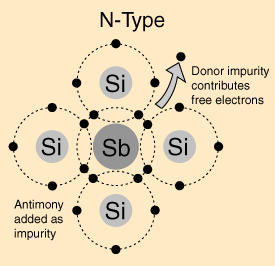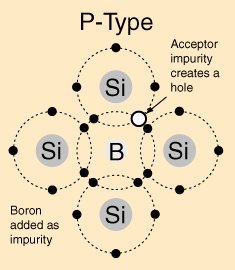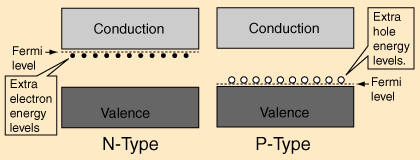African Virtual University

(a)

(b)

Figure 1.4 (a) N-type semiconductor formed adding antimony to Si

(b) P-type semiconductor formed by adding boron to Si

The donor atom becomes a positively-charged ion after giving away one of its va-

lence electron, but it can not take part in conduction because it is firmly fixed into

the crystal lattice.

Addition of antimony greatly increases the number of conduction electrons. Thus

the concentration of electrons in conduction band is increased and exceeds the

concentration of holes in the valence band. In this situation, we see that in the N-type

semiconductors electrons are the majority carriers, while holes constitute the minority

carriers. When the number of charge carriers in the conduction band increases, the

Fermi level shifts upwards towards the conduction band as shown in Fig 1.5(b).

(a)

(b)

Figure 1.5 Showing relative positions of the Fermi level to conduction bandAfrican Virtual University

Activity 1.5

P-type Extrinsic Semiconductor

Here you learn that:

P-type extrinsic semiconductor is formed when a trivalent atom like boron is added

to pure germanium crystal (or pure silicon crystal as shown in Fig. 1.4(b).

The three valence electrons of boron atom form covalent bonds with four surrounding

silicon atoms, but one bond is left incomplete. This gives rise to a hole.

The acceptor impurity produces as many positive holes in silicon crystal as there

are boron atoms and thefore a P-type (P for positive) extrinsic semiconductor is

formed.

In the P-type semiconductor, conduction is the movement of holes in the valence

band.

Holes form the majority carriers whereas electrons constitute minority carriers.

Unlike in the N-type semiconductor, the Fermi level in P-type shifts towards the

valence band, Fig 1.5(a) because, the majority carriers which are the holes are found

in the valence band.

Activity 1.6

Conductivity of intrinsic Semiconductors

In an intrinsic semiconductor the total current, I , is due to the sum of electrons flow

and hole flow. This is given by Eq .

I = I +I

e

h

(7)

From equation (7), it can be shown that

(i)

I = ne μ + μ AV

i ( e

h )

/ l

(8)

1

(ii) ρ =

τ

n e(

) ohm− m

(9)

μ + μ

i

e

h

(iii) C urrent density, J

e(

)E

E

(10)

= n μ + μ

= σ

i

e

h

i

where A is cross section area of semiconductor,V is voltage across its length, l , E

is the electric field. The other symbols have their usual meanings.African Virtual University

Task 1.6.1 Note making and verfications of equations

(a) Use the available references and verify Eqs. (8), (9) and (10)

Hint: Note that in an intrinsic semiconductor, n = p (the number of holes).

i

i

Activity 1.7

Conductivity of Extrinsic Semiconductor

In extrinsic semiconductors, current density, J, is given by Eqs. (11) and (12).

(i) For N-type semiconductor J

(

)E . (11)

= e n μ + p μ

n

n e

n h

(ii) For P- type semiconductor J = e n μ + p μ

(

)E . (12)

p

p e

p h

where n ,and are electron and hole densities in the N- type semiconductor af-

n

pn

ter doping and n and p are electron and hole in the P- type semiconductor after

p

p

doping.

You need to try solving many numerical problems related to activity 1.6 in order

to develop sufficient confidence in this topic

Activity 1.7.2 Drifts

In this activity you will learn that:

Directed motion of charge carriers in semiconductors occurs through two mecha-

nisms:

i. Charge drift under the influence of applied electric field and

ii. Diffusion of charge from a region of high charge density to one of low charge

density.

When an electric field is applied to a crystal, the charge carriers attain a direct motion

which results into a net average velocity called drift velocity, v, in the direction of the applied electric field, E and produces a current. The relation between v and E is:

v = μE

(13)

μ is mobility.African Virtual University

The total current density due to electron and hole drift is

J = J + J = eμ nE + eμ pE = e(nμ + pμ )E

e

h

e

h

e

h

(14)

where n and p are electron density and hole density respectively.

Activity 1.7.3: Diffusion

The major concepts that you learn in this section are:

(i) Diffusion is a gradual flow of charge from a region of high density to a region

of low density which eventually leads to an electric current without an applied

field bieng applied.

(ii) The diffusion of carriers is proportional to the carrier density gradient, and

the diffusion constant or diffusion coefficient D which has a unit of m2 / s .

(iii) Current density due to hole diffusion is

dp

J = −eD

. and

(15)

h

h dx

Similarly, current current density due to electron diffusion is.

dn

J = −eD

.

(16)

e

e dx

Where D ,D = electron and hole diffusion constants respectively

e

h

dn

dx

dp

dx

For simulation of diffusion of how the Fermi level varies with carrier concentration

see: http://jas.eng.buffalo.edu/education/semicon/fermi/bandAndLevel/index.html.

http://jas.eng.buffalo.edu/education/semicon/fermi/bandAndLevel/index.html 10th

October 2007.African Virtual University

Activity 1.7.4 Combined Drift and Diffusion currents

Drift and diffusion processes may be present simultaneously in semiconductors, thus